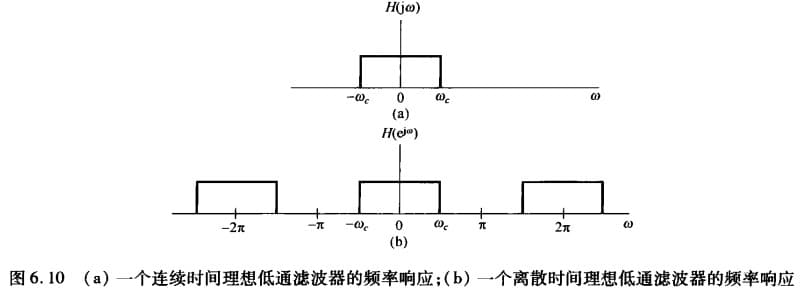# 理想频率选择滤波器

$\newcommand{\dif}{\mathop{}\!\mathrm{d}} \newcommand{\xleftrightarrow}{\stackrel{#1}{\longleftrightarrow}} \newcommand{\F}{\mathcal{F}} \newcommand{\ft}{\xleftrightarrow{\F}}$

# 滤波器

1. 滤波成形
2. 滤波选择$H(j\omega)= \begin{cases} 1 & |\omega|\leq\omega_c\\ 0 & |\omega|>\omega_c \end{cases}$

$h(t)=\frac{\sin \omega_c t}{\pi t}=\frac{\omega_c}{\pi} \mathrm{Sa}(\omega_c t)$